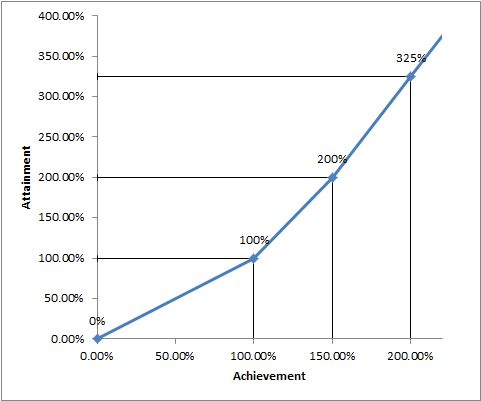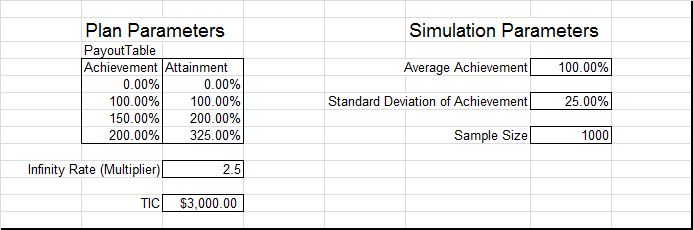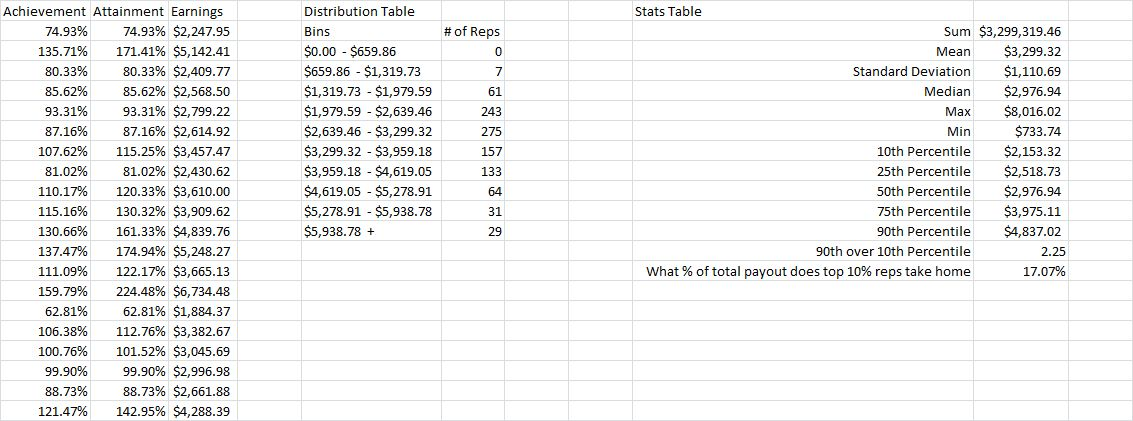# Modeling a simple compensation plan in Python

09 January 2014

## IntroductionOver the past few years python has grown into a serious scientific computing language. This owes itself to several mathematical packages like numpy, scipy and pandas maturing and being trusted by the scientific community. These packages and the inherent simplicity of python has had a democratizing effect on the ability to perform complex mathematical modeling without expensive proprietary software.

In this blogpost I wish to utilize these techniques that is commonplace in the scientific community to try and apply it to the sales compensation/analytics domain. I'm going to attempt to model a basic sales compensation plan using numpy and python. The intent is to keep the model simple and to get it up and running fast. Future blogposts will introduce more complex elements and more advanced modeling techniques. The hope is that this basic model will demonstrate the power of these python-based modeling techniques and its simplicity.

## The Interface

Firstly, let's settle on an input/output interface. I believe business users are still some way off from setting parameters/data in formats like JSON/XML/YAML. This eliminates those possibilities. As most of us are used to Excel (more than we like to admit), I'd like to keep the input/output interface as Excel. I'd like the model to read inputs and parameters from Excel and write the final results into an output sheet on Excel. Python luckily has an excellent package called openpyxl that allows it to interface with an Excel file (Excel 2007 onwards only). We'll use this package to read our inputs and parameters and to write the outputs of our model. DataNitro and Pyxll are two other python packages you can use for interfacing with Excel, however they run as an addin to Excel rather than as an independent python package.

## The Plan

I've chosen a very simple Achievement vs Attainment Payout Table for purposes of this model. I've created a named range "PayoutTable" in my excel file (named "plan_modeling.xlsx") containing this payout table.

I'm going to assume a simple payout table with linearly interpolated attainment between two subsequent performance levels. Below is a graphical look at my payout curve. The slope of the line above the highest performance level defined in the table is determined by the "Infinity Rate" ParameterThe Target Incentive Dollars for the given class of reps is defined using the "TIC" parameter

Earnings for the plan will be calculated as the attainment as calculated using the payout table based on the achievement times the Target Incentive.

## Simulation Parameters

To simulate performance of a salesforce on this plan I'm going to use a normal distribution of achievements around some mean and standard deviation. These parameters are defined in my Excel file as "AverageAchievement", "StdDevAchievement". The sample size of the population of sales reps that I use to model this plan is defined by the "SampleSize" parameter. Overall the plan and simulation parameters section looks like the following## Model Setup

Alright! Time to write some code!!

First let's import the required packages. Numpy and openpyxl

```import numpy
import openpyxl
from openpyxl.style import NumberFormat
```

I've created two helper packages. openpyxl_helpers has methods for frequently used interactions with the excel file using the openpyxl library. CompUtils sets up a Rate_Table class to maintain a payout table and has a method to apply the rate table to an achievement distribution

```import CompUtils
from openpyxl_helpers import *
```

Open the workbook using openpyxl

```# Open workbook
```

Pull in all the plan and simulation parameters from the excel file using their named ranges.

```# Read plan parameters

```

Next, we'll initialize the Rate_Table class from our helper package CompUtils. We'll add all rows from the payout_table_range that we read from the excel file and then set the infinity rate parameter. The Rate_Table class expects the lookup field to be called 'start' and the value field to be called 'base'. Since we've used 'achievement' and 'attainment' instead, we'll override those names by passing startKey and baseKey.

```# Initialize Rate Table
payout_table = CompUtils.Rate_Table()
payout_table.set_infinity_rate(infinity_rate)
```

Finally, all our parameters have been retrieved, our rate table has been set up. Now wee're ready to do some modeling. Let's first generate the achievement sample using our simulation parameters. We'll use the numpy.random.normal function.

```# Populate the achievement sample
achievement_distribution = numpy.random.normal(average_achievement,std_achievement,sample_size)
```

Now calculate the attainment for this achievement sample by applying the rate table to it.

```# Calculate Attainment based on the Payout Table
attainment = CompUtils.calculate_lookup_attainment(achievement_distribution,payout_table.rows)
```

Calculate earnings by multiplying the attainment values against TIC. Note that the attainment is a numpy.ndarray and is being multiplied by a scalar TIC. Numpy allows us to do such multiplications simply and it inherently understands how to handle such an operation.

```# Calculate Earnings as Attainment times Target Incentive
earnings = attainment * TIC
```

And that's it. We've already calculated earnings for all reps in the sample. And it took all of 3 lines of code for the full model!! Now let's write some of our results out into excel.

```# Create an output sheet for us to write to
output_ws = wb.create_sheet(title='Output')

# Write Achievement, Attainment and Earnings into columns
write_list_of_values(output_ws,'A1',list(achievement_distribution),'Achievement',number_format=NumberFormat.FORMAT_PERCENTAGE_00)
write_list_of_values(output_ws,'B1',list(attainment),'Attainment',number_format=NumberFormat.FORMAT_PERCENTAGE_00)
write_list_of_values(output_ws,'C1',list(earnings),'Earnings',number_format=NumberFormat.FORMAT_CURRENCY_USD_SIMPLE)
```

That gave us the raw values of the achievement sample, attainment and earnings of that sample. Now let's create some more useful analytical tables. The openpyxl_helpers package has a couple of predefined methods to help create a quick distribution table and some statistics in a stats table. Let's add those into our output sheet now.

```# Create a Distribution Table
create_distribution_table(output_ws,'E1',earnings,number_format=NumberFormat.FORMAT_CURRENCY_USD_SIMPLE)

# Create a Stats Table
create_stats_table(output_ws,'I1',earnings,number_format=NumberFormat.FORMAT_CURRENCY_USD_SIMPLE)
```

Finally let's write the output to a new file

```# Write the output to a new file
wb.save(filename='/home/sujeet/Desktop/plan_modeling_simulated.xlsx')
```

Our newly generated output file should have a new sheet called "Output" with data like the below screenshot. The stats table gives us some useful metrics and the distribution table can be used to plot a bell-curve of the reps earnings.## The tip of the iceberg

This is a very basic model. My intent was only to display how painless it is to use python, numpy, etc. to create a basic sales compensation/analytics model. This code can be adapted to perform analysis on a complex model, just as easily. Imagine the potential in quota setting models, territory alignments, call planning, etc.

You can get the code for this whole model including the helper packages at sujeetpillai/simple_python_comp_model

Incentius can help create, maintain and tweak such models to achieve your business goals. This is a zone that we're very excited about and can't wait to help you realize the potential for such techniques!

How did you like this blogpost? Let us know in the comments below or on our social media pages.

If you got this far, we think you’d like our future blog content, too. Please subscribe on the right side.